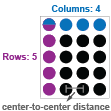# generateCircleGridPoints

Generate circle grid point locations

## Syntax

``worldPoints = generateCircleGridPoints(patternDims,centerDistance)``
``[worldPoints] = generateCircleGridPoints(___,PatternType="symmetry")``

## Description

example

````worldPoints = generateCircleGridPoints(patternDims,centerDistance)` generates circle grid point locations based on the specified number of circles in each dimension `patternDims` and the specified distance in world units between the centers of two adjacent circles `centerDistance`.For more details on circle grid patterns, see Circle Grid Patterns.```
````[worldPoints] = generateCircleGridPoints(___,PatternType="symmetry")` specifies the type of circle grid for which the function generates point locations. Specify `PatternType` as `"asymmetric` or `"symmetric"`. By default, the function generates point locations for an asymmetric circle grid.```

## Examples

collapse all

Specify the distance between the centers of adjacent circles, in millimeters (mm). By default, the function generates point locations for an asymmetric circle grid.

```centerDistance = 9; worldPoints = generateCircleGridPoints([4 11],centerDistance);```

Specify the distance between the centers, in millimeters (mm). Set the pattern type to `"symmetric"`.

```centerDistance = 19; worldPoints = generateCircleGridPoints([8 11],centerDistance, ... PatternType="symmetric");```

## Input Arguments

collapse all

Pattern dimensions, specified as a 1-by-2 vector that represents the number of circles in each dimension. For the asymmetric pattern, Dimension 1 corresponds to the number of circles in each column. Dimension 2 corresponds to the total number of circles in the first two adjacent rows. For more details on circle grid patterns, see Circle Grid Patterns.

AsymmetricSymmetricDistance between centers of two adjacent circles in the same row or column, specified as a scalar in world units, such as millimeters.## Output Arguments

collapse all

Circle grid center locations, returned as an M-by-2 matrix. Each row of the matrix specifies the xy-coordinates of a circle center relative to the origin. The origin (0,0) is the center of the top-left circle in the grid. M is the total number of circles in the grid,calculated as the product of the circle count in each dimension: `prod`(`patternDims`).

Introduced in R2021b